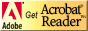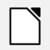# PC221 Analog Electronics I

## AC Measurements

### Objectives

1. To review the use of the function generator.
2. To introduce measurement of simple AC circuits.

### Equipment

• digital multimeter, bench power, function generator, oscilloscope

### Background

Analyzing circuits with both DC and AC components is slightly more complicated than analyzing DC circuits. One reason is because of the challenge involved in measuring AC quantities.

#### Amplitude, Peak-to-peak, or RMS?

A critical issue in AC measurements is terminology; values can be described in at least 3 different ways. You always need to know which is being used.
AC voltages can be given as

• amplitude
• peak-to-peak
(i.e. twice the amplitude)
• RMS

The last one, RMS, is the most tricky because it depends on the shape of the waveform.
Question: How would the RMS for a triangle wave be related to the RMS of a sawtooth wave? Be prepared to explain this.

Most digital meters have separate scales for DC and AC measurements.

### Procedure

For the following test, make sure the coupling for the oscilloscope channel(s) is set to DC.

To test whether the digital meter measures RMS or amplitude, do the following:

1. Connect the signal generator to the oscilloscope and the digital meter.

2. Read the information about measuring AC voltage with a digital multimeter.

3. Produce a 1 kHz, one volt sine wave with no DC offset and record the offset and amplitude from the oscilloscope in Table 1.
Since the offset is midpoint voltage of the wave, it is the average of the voltages at the top and bottom of the wave.
Since the amplitude is half of the peak-to-peak value, it is the difference between the voltages at the top and bottom of the wave, divided by two.

4. Measure the voltage using both the DC and AC settings on the meter. What does this tell you?

Shape Table 1: Voltage Measurements (no offset) Sine Square Triangle

5. Repeat the measurements for the other shapes listed in Table 1.

7. Measure the frequency of the waveform using both the meter and the oscilloscope.

Shape Table 2: Frequency Measurements Sine Square Triangle

8. Adjust the DC offset on the signal generator, and see how the DC and AC meter readings change.

9. Now change the coupling for the oscilloscope to AC. Adjust the DC offset on the signal generator, and see what happens to the signal on the oscilloscope.

For a specific value of offset, fill in Table 3.

Shape Table 3: Voltage Measurements (with offset) Offset (volts) Sine Square Triangle

Can you measure the amplitude of the signal in AC mode? Can you measure the offset in AC mode?

It is critical that you understand what AC and DC coupling do. Specifically, you need to understand that it is wrong to say that you should use DC coupling for measuring DC voltages and AC coupling for AC voltages. DC coupling is always a better choice as long as it makes the AC portion of the signal large enough to measure on the screen.

Switch the coupling back to DC.

10. Once you feel you know how to convert between meter readings and oscilloscope readings, switch the waveform; first to a square wave and then to a triangle wave to see how that affects the meter readings.
Make sure to keep the waveform the same size, so that the amplitude and peak-to-peak measurements should stay the same.

11. Sketch the waveforms for a sine wave, a triangle wave, and a square wave of the same amplitude and write what the digital meter reads for each on the sketch, on both the DC and AC settings.

12. What do your results tell you about using a digital meter for AC signals when you don't know what the waveform looks like? Why is an oscilloscope a better choice?

13. Does the AC reading on your digital meter display amplitude, peak-to-peak, or RMS voltage?
For a sine wave, how would you convert an RMS reading to amplitude?
For a sine wave, how would you convert an amplitude reading to RMS?

Explain your findings to the lab instructor.

## ResourcesIf you need to update a browser, you might try Firefox which is
• free
• open source
• available for several platformsSince this page uses cascading style sheets for its layout, it will look best with a browser which supports the specifications as fully as possible.

If you are looking for an office package, with a word processor, spreadsheet, etc., you might try LibreOffice which is
• free
• open source
• available for several platformsGo to the main page for the Department of Physics and Computer Science.

Wilfrid Laurier University